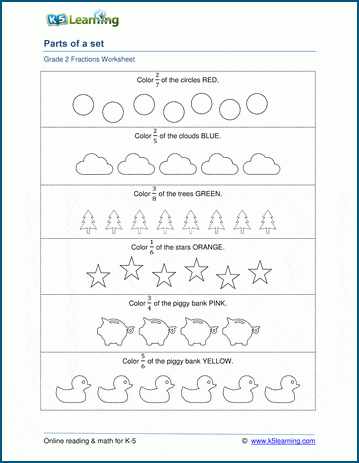# Coloring fractions of a group

## Understanding fractions as part of a group

These worksheets emphasize an understanding of fractions as part of a set or group (as opposed to as part of a whole divided into equal parts).  Students are given a group of objects and asked to color a fraction of them.## More fractions worksheets

Explore all of our fractions worksheets, from dividing shapes into "equal parts" to multiplying and dividing improper fractions and mixed numbers.

## What is K5?

K5 Learning offers reading and math worksheets, workbooks and an online reading and math program for kids in kindergarten to grade 5.  We help your children build good study habits and excel in school.